Next: 1.2 Influence of the Up: 1. Introduction Previous: 1. Introduction

# 1.1 Grid Requirements for Numerical Discretization Methods

Therefore, numerical methods have to be applied. Typically, a mesh is spanned over the simulation domain and a numerical solution is sought, which approximates the solution in the sampling points. The calculation is done by discretizing the differential equations by the methods of Finite Differences , Finite Elements  or Finite Boxes . Usually this discretization results in a large equation system, which has to be solved. Each differential equation typically delivers an equation system with the rank of the system being in the same size as the number of grid points. Normally the given differential equations are not separable and in sum, an equation system with the number of unknowns being equal the number of differential equations multiplied with the number of grid points must be solved. For nonlinear systems, the final solution can only be found iteratively by applying Newton's method . For time dependent problems, the solution of each time-step has to be calculated iteratively until the desired point of time is reached. For implicit methods each time-step requires the solution of the equation system. Therefore, these iterations can be very expensive in terms of calculation time, reaching the maximum in nonlinear time-variant problems.

Ideally the mesh generation process and the simulation process should be separated. This procedure eases the development of the simulation tools and guarantees the modularity and flexibility of the tools. However, these steps are not necessarily separable. For one-dimensional problems, the methods of Finite Differences, Finite Elements (assuming linear Ansatzfunctions) and Finite Boxes (also known as Box Integration) result in the same solution. Within two-dimensional simulations, Finite Difference schemes exist in practicable form only for ortho-product grids where they deliver the same equation-system as for Finite Boxes. Moreover, Finite Elements and Finite Boxes also deliver the same solution. However, in three dimensions discretization becomes more difficult and Finite Boxes and Elements differ in their solution .

Additionally the different simulation methods have different demands on the grid. In two dimensions, these criteria lead to the same geometrical grid criteria. However, for three-dimensional problems, different criteria have to be fulfilled, depending on the simulation method. On the one hand, Box Integration requires the well-known Delaunay grids, and on the other hand, Finite Element analysis should be based on a grid fulfilling a Cotangent Criterion'', where many questions for grid generation are still unresolved. Details of the discretization process and the resulting grid criterions will be shown in Section 2.2. That is the reason why good Box Integration grids will not necessarily be good Finite Element grids .

Finally, a specifically designed grid adaptation method can dramatically reduce the calculation effort and the memory consumption. These specially adapted grids require less grid points and produce more compact equation systems for reaching the same accuracy. Even within one equation class, the requirement on the grid can vary dynamically. A simple example, frequently used within electrical device manufacturing, is a diffusion simulation of implanted dopants. This problem requires a transient simulation where a relatively sharp implanted dopant profile diffuses to a smooth profile over time. To achieve accurate simulation results the grid density must be high in areas with high concentration curvatures . In addition, locations that require high grid-resolution will move within the material regions during diffusion time. While moving, the dopant distribution is also smoothened (except for some special applications). This leads to the requirement of adaptive mesh refinement. In view of calculation times, this refinement cannot be performed by frequent regridding. Usually an approach of hierarchical refinement and coarsening will be chosen (see Section 2.15).

Basically, an overall increase of sampling points will also increase the accuracy of the simulation (until numerical instabilities limit a continuative increase of sampling points ). Despite the limited machine resources, namely CPU time and memory, this fact cannot be exploited indefinitely. Consider the case where a two-dimensional grid with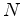grid points is expanded to three dimensions. Based on the consideration that the two-dimensional grid is spanned by about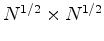grid points, the estimated number of points of the three-dimensional grid will lead to an increase of computation points to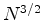. The increase of memory consumption is of the same magnitude, not including the storage required for the third coordinate values of the grid points plus additionally used point references of the grid elements. The computation times will rise by the same amount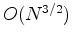for the best fitted solvers available like multigrid solvers, which have nearly linear complexity,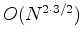for solvers which account for the sparsity of the equation systems, like Conjugate Gradient solvers, and up to an amount of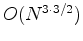for simple Gaussian solvers. The limited calculation accuracy of the floating-point units of the simulation machines also poses a limit to the equation system size.Next: 1.2 Influence of the Up: 1. Introduction Previous: 1. Introduction

J. Cervenka: Three-Dimensional Mesh Generation for Device and Process Simulation